# Experiment templates and LaTeX typesetting

### Experiment templates

All experiment templates are licensed under the Creative Commons Attribution-Non Commercial 4.0 International (CC BY-NC 4.0) license.Simple reading time experiment template for SR Research EyeLink 1000. Participants view a fixation point in the middle of the first letter of the sentence. After fixating the point, the whole sentence appears, as well as a second fixation point. When participants fixate a second fixation point or read the sentence for longer than 20000 ms, the sentence disappears. Some sentences are followed by a comprehension question with two answer alternatives.

This is an experiment template in which participants read sentences in segments and occasionally have to answer a comprehension question. The experiment consists of the following parts: Obligatory ethics agreement, form for inputting participant information, instructions, exercise, main experiment sequence, end screen and experiment explanation.

#### Online questionnaire experiment: acceptability judgments

An online questionnaire experiment. Participants read a sentence and are simultaneously presented with a 7-point Likert scale. They must to rate how natural the sentence sounds to them before proceeding to the next sentence.

#### Online questionnaire experiment: forced choice

An online questionnaire experiment. Participants read a sentence and are simultaneously presented with a forced-choice question with two answer alternatives. They must choose one of the answers in order to proceed to the next sentence.

#### In Lab experiment: self paced reading

A self-paced reading experiment template with a moving window paradigm. Participants view a fixation point in the middle of the screen. Then the sentence appears with the letters replaced by underscores. Participants press the space bar in order to reveal a sentence segment. After having pressed the space bar again, the segment disappears and the subsequent segment appears. This continues until the last segment in the sentence. Some sentences are followed by a comprehension question with two answer alternatives. This experiment contains a script by Jan Anderssen (jan[at]linguist.umass.edu).

#### Citation

These templates can be cited as e.g.:

Prysłopska, Anna. (2021) Eye-tracking during reading experiment template. https://pryslopska.com/experiments.html, version 1.0. Accessed: 20XX-XX-XX.

BibTeX citation

@misc{pryslopska2021eyetracking, title = {Eye-tracking during reading experiment template}, author = {Prysłopska, Anna}, howpublished = \url{https://pryslopska.com/experiments.html}, year = {2021}, version = {1.0}, note = {Accessed: 20XX-XX-XX} }

### Typesetting

I've spent lots of time trying to get my thesis to look right and recreating some more and less famous figures. Below are some figures that I had to make from scratch and would have appreciated if someone had already provided the code. If you think you can make them better, please tell me so I can improve. You will need XeLaTeX and Tikz for this.

#### Overview of common eye-tracking measures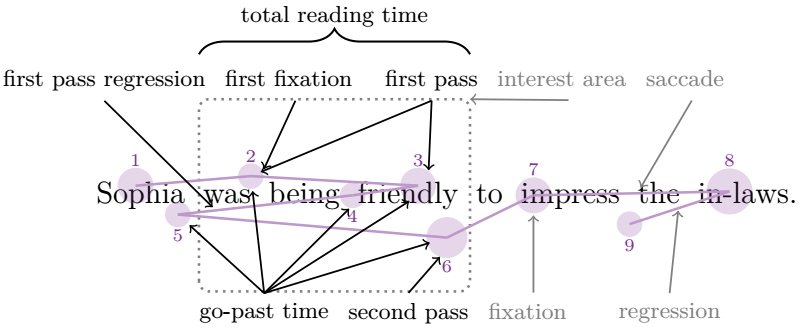A depiction of common eye-tracking measures (total reading time, first pass regression, first fixation duration, first pass reading time, go-past time, second pass reading time), as well as a single fixation, regression, saccade, and interest area. Please, cite this figure as:
Prysłopska, Anna (2021). Being Friendly is Difficult: Psycholinguistic Experiments on Agentivity in Copular Constructions. PhD thesis, University of Tübingen.

\documentclass[class=article]{standalone} \usepackage{tikz} \usetikzlibrary{decorations.pathreplacing, positioning} \definecolor{darkpurple}{RGB}{123,50,148} \begin{document} \tikzset{every picture/.style={line width=1pt}} \tikzset{arrow/.style={line width=0.7pt}} \tikzset{circle/.style={draw, draw opacity=0, fill=darkpurple, fill opacity=0.2}} \tikzset{numbers/.style={text=darkpurple, font=\scriptsize}} \tikzset{annotation/.style={text=black, font=\small}} \begin{tikzpicture}[x=0.75pt,y=0.75pt] % BOX \draw[draw=gray, rounded corners, dotted, line width=1pt, opacity=1] (-254,-50) rectangle ++(141,100); % SENTENCE \draw (-125,0) node [font=\large] {\fontdimen2\font=7pt Sophia was being friendly to impress the in-laws.}; % CIRCLES \draw [circle] (-287,5) circle (.7em) node (1){}; \draw [circle] (-227,10) circle (0.5em) node (2){}; \draw [circle] (-140,5) circle (0.7em) node (3){}; \draw [circle] (-174,0) circle (0.5em) node (4){}; \draw [circle] (-265,-10) circle (0.5em) node (5){}; \draw [circle] (-125,-22) circle (0.8em) node (6){}; \draw [circle] (-80,0) circle (0.7em) node (7){}; \draw [circle] (22,2) circle (0.9em) node (8){}; \draw [circle] (-30,-15) circle (.5em) node (9){}; % TEXT \draw [numbers] node [above = 0 em of 1, anchor=south] {1}; \draw [numbers] node [above = -0.2em of 2, anchor=south] {2}; \draw [numbers] node [above = -0em of 3, anchor=south] {3}; \draw [numbers] node [below = -0.2em of 4, anchor=north] {4}; \draw [numbers] node [below = -0.2em of 5, anchor=north] {5}; \draw [numbers] node [below = 0.1em of 6, anchor=north] {6}; \draw [numbers] node [above = 0em of 7, anchor=south] {7}; \draw [numbers] node [above = .2em of 8, anchor=south] {8}; \draw [numbers] node [below = -0.2em of 9, anchor=north] {9}; \draw [annotation] node [below = 5 em of 7, anchor=south] (fixation) {\textcolor{gray}{fixation}\phantom{g}}; \draw [annotation] node [left = 0 em of fixation] (SPRT) {second pass}; \draw [annotation] node [left = 0 em of SPRT] (RPD) {go-past time}; \draw [annotation] node [right = 0.8em of fixation] (regression) {\textcolor{gray}{regression}}; \draw [annotation] node [above = 7.5em of SPRT, xshift=0.9em] (FPRT) {first pass}; \draw [annotation] node [left = 0em of FPRT] (FF) {first fixation\phantom{p}}; \draw [annotation] node [left = 0em of FF] (FPR) {first pass regression}; \draw [thick, decorate, decoration={brace,amplitude=10pt}, yshift=2.5em] (-254,40) -- (-113,40) node [black,midway,yshift=1.5em] (TRT) {\small total reading time}; \draw [annotation] node [right = 0 em of FPRT] (IA) {\textcolor{gray}{interest area}\phantom{p}}; \draw [annotation] node [right = -0.5 em of IA] (saccade) {\textcolor{gray}{saccade}\phantom{p}}; % LINES \draw [color=darkpurple!50] (1.center) -- (2.center) node [midway] (A) {} ; \draw [color=darkpurple!50] (2.center) -- (3.center) ; \draw [color=darkpurple!50] (3.center) -- (4.center) node [midway] (C) {} ; \draw [color=darkpurple!50] (4.center) -- (5.center) node [midway] (D) {} ; \draw [color=darkpurple!50] (5.center) -- (6.center) ; \draw [color=darkpurple!50] (6.center) -- (7.center) ; \draw [color=darkpurple!50] (7.center) -- (8.center) node [midway] (E) {} ; \draw [color=darkpurple!50] (8.center) -- (9.center) node [midway] (B) {} ; % ARROWS \draw [->, draw=gray, arrow, shorten >=.4em] (fixation) -- (7.south); \draw [->, draw=gray, arrow, shorten >=.7em] (saccade.south) -- (E.south); \draw [->, draw=gray, arrow, shorten >=0em] (IA.south) -- (-113,50); \draw [->, draw=gray, arrow, shorten >=.5em] (regression.north) -- (B.north) ; \draw [->, arrow, shorten >=0em] (FPRT.south) -- (2); \draw [->, arrow, shorten >=.6em] (FPRT.south) -- (3.east); \draw [->, arrow, shorten >=1em] (SPRT.north) -- (6.east); \draw [->, arrow, shorten >=.2em] (RPD.north) -- (2); \draw [->, arrow, shorten >=.4em] (RPD.north) -- (3.south); \draw [->, arrow, shorten >=.2em] (RPD.north) -- (4.south); \draw [->, arrow, shorten >=.1em] (RPD.north) -- (5); \draw [->, arrow, shorten >=.3em] (RPD.north) -- (6); \draw [->, arrow, shorten >=.3em] (FF.south) -- (2.east); \draw [->, arrow, shorten >=.3em] (FPR.south) -- (-244,-9); \end{tikzpicture} \end{document}

#### Eye-tracking trial structure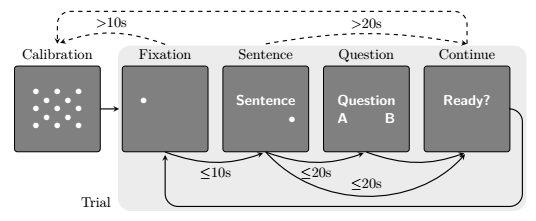An outline of the procedure in a single eye-tracking trial. Please, cite this figure as:
Prysłopska, Anna (2021). Being Friendly is Difficult: Psycholinguistic Experiments on Agentivity in Copular Constructions. PhD thesis, University of Tübingen.

\documentclass[class=article]{standalone} \usepackage{tikz} \usetikzlibrary{backgrounds, calc, positioning} \begin{document} \tikzset{block/.style={rectangle, rounded corners=2, draw, fill=gray, minimum width=6em, minimum height = 6em, align=center, font=\small\bfseries\sffamily, text=white}} \begin{tikzpicture}[node distance = 1.5em, arrow/.style = {thick,-stealth}] % Boxes \node [block] (calibration) { $\bullet$\hspace{1em}$\bullet$\hspace{1em}$\bullet$\\ $\bullet$\hspace{1em}$\bullet$\hspace{1em}$\bullet$\\ $\bullet$\hspace{1em}$\bullet$\hspace{1em}$\bullet$}; \draw ($(calibration.north)+(0,-0.85)$) node {\textcolor{white}{$\bullet$\hspace{1em}$\bullet$}}; \draw ($(calibration.south)+(0, 0.85)$) node {\textcolor{white}{$\bullet$\hspace{1em}$\bullet$}}; \node [block, right = of calibration] (point) {$\bullet$\hspace*{3em}\\}; \node [block, right = 1em of point] (sentence) {Sentence\\ \hfill$\bullet$}; \node [block, right = 1em of sentence] (question) {Question\\ A \hfill B}; \node [block, right = 1em of question] (next) {Ready?\\}; % Descriptions \node [above = 0.1em of calibration] (cal) {Calibration}; \node [above = 0.1em of point] (fix) {Fixation}; \node [above = 0.1em of sentence] (sen) {Sentence}; \node [above = -0.1em of question] (que) {Question}; \node [above = 0.1em of next] (nex) {Continue}; % Arrows %% Straight \draw [arrow, shorten >= 2pt] (calibration) to (point); %% Bendy below \draw [arrow, shorten >= 2pt] (point.south) to [bend right=20] node[midway,below] {$\leq$10s} (sentence.south); \draw [arrow, shorten >= 2pt] (sentence.south) to [bend right=20] node[midway,below] {$\leq$20s} (question.south); \draw [arrow, shorten >= 2pt] (sentence.south) to [bend right=48] node[midway,above] {$\leq$20s} (next.south); \draw [arrow, shorten >= 2pt] (question.south) to [bend right=20] (next.south); \draw [arrow, rounded corners=9, shorten >= 2pt] (next.east) -- ++(0.3,0) -- ++(0,-2.4) -| (point.south); %% Bendy above \draw [arrow, dashed, shorten >= 2pt] (fix.north) to [bend right=20] node[midway,above] {>10s} (cal.north); \draw [arrow, dashed] (sen.north) to [bend left=10] node[midway,above] {>20s} (nex.north); \draw [arrow, dashed, rounded corners=9] (nex.north) -- ++(0,0.8) -| (cal.north); % Trial marking \begin{scope}[on background layer] \draw[draw=none, rounded corners=9pt,fill=gray!15] ($(point.north west)+(-0.1,0.5)$) rectangle ($(next.south east)+(0.4,-1.45)$); \node [below = 2.8em of calibration, xshift=2.7em] (trial) {Trial}; \end{scope} \end{tikzpicture} \end{document}

#### Ontology of kinds, objects, and stages (Carlson, 1977)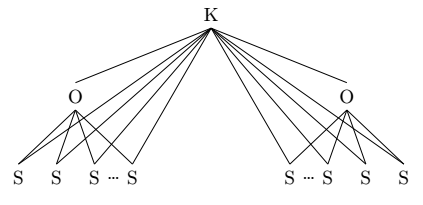Carlson GN (1977). Reference to Kinds in English. Ph.D. thesis, University of California.

\documentclass[class=article]{standalone} \usepackage{tikz-qtree} \begin{document} \begin{tikzpicture}[level distance=1.5cm, level 1/.style={sibling distance=5cm}, level 2/.style={sibling distance=0.7cm}] \node (a) {K} child {node {O} child {node (b) {S}} child {node (c) {S}} child {node (d) {S}} child {node (e) {S}} } child {node {O} child {node (f) {S}} child {node (g) {S}} child {node (h) {S}} child {node (i) {S}} }; \path (d) -- (e) node [midway] {\ldots}; \path (f) -- (g) node [midway] {\ldots}; \path[every node/.style={font=\sffamily\small}] (a.south) edge node {} (b.north) (a.south) edge node {} (c.north) (a.south) edge node {} (d.north) (a.south) edge node {} (e.north) (a.south) edge node {} (f.north) (a.south) edge node {} (g.north) (a.south) edge node {} (h.north) (a.south) edge node {} (i.north); \end{tikzpicture} \end{document}

#### Raising and control Infl (Diesing, 1992)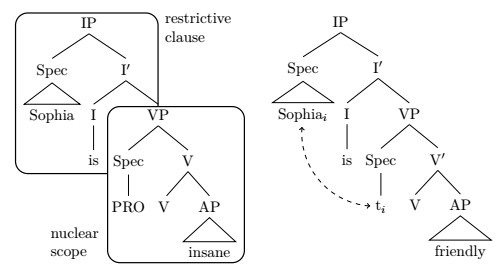Diesing M (1992). Indefinites. MIT press.

\documentclass[class=article]{standalone} \usepackage{tikz-qtree} \usetikzlibrary{calc, backgrounds} \begin{document} \begin{tikzpicture} \tikzset{every tree node/.style={align=center, anchor=north}} \Tree [.\node(ip){IP}; [.{Spec} \edge[roof]; \node(subj){Sophia}; ] [.\node(ibar){I$'$}; [.\node(i){I}; \node(aux){is};] [.\node(vp){VP}; [.\node(specvp){Spec}; \node(subj-t){PRO}; ] [.V$’$ \node(v){V }; [.AP \edge[roof]; \node(adj){insane}; ]]]]]]] \begin{scope}[on background layer] \draw[thick, rounded corners=8pt,fill=white] ($(subj.north west)+(-0.2,2.1)$) rectangle ($(vp.south east)+(-0.38,-1.1)$); \node[yshift=-1em, xshift=-1.8em, text width=2cm] at (current bounding box.north east) {restrictive clause}; \draw[thick, rounded corners=8pt,fill=white] ($(specvp.north west)+(0,1)$) rectangle ($(adj.south east)+(0.2,0)$); \node[yshift=1.3em, xshift=5.2em,text width=2cm] at (current bounding box.south west) {nuclear scope}; \end{scope} \end{tikzpicture} ~ \begin{tikzpicture} \Tree [.\node(ip){IP}; [.Spec \edge[roof]; \node(subj){Sophia$_i$}; ] [.\node(ibar){I$'$}; [.\node(i){I}; \node(aux){is};] [.\node(vp){VP}; [.\node(specvp){Spec}; \node(subj-t){t$_i$} ;] [.\node(vbar){V$'$}; \node(v){V}; [.AP \edge[roof]; \node(adj){friendly};] ] ] ] ] \draw[dashed, <->] (subj-t) [in=-90, out=-90, bend left=45] to (subj); \end{tikzpicture} \end{document}

#### Copular small clause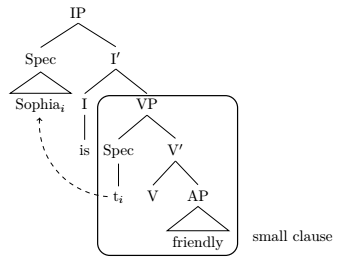A tree diagram of the elements of a copular clause.

\documentclass[class=article]{standalone} \usepackage{tikz-qtree} \usetikzlibrary{calc, backgrounds} \begin{document} \begin{tikzpicture} \Tree [.\node(ip){IP}; [.Spec \edge[roof]; \node(subj){Sophia$_i$}; ] [.\node(ibar){I$'$}; [.\node(i){I}; \node(aux){is};] [.\node(vp){VP}; [.\node(specvp){Spec}; \node(subj-t){t$_i$} ;] [.\node(vbar){V$'$}; \node(v){V}; [.AP \edge[roof]; \node(adj){friendly};] ] ] ] ] \begin{scope}[on background layer] \draw[thick, rounded corners=8pt,fill=white] ($(specvp.north west)+(0,1)$) rectangle ($(adj.south east)+(0.2,0)$); \node[yshift=1.3em, xshift=3.8em,text width=2cm] at (current bounding box.south east) {small clause}; \end{scope} \draw[dashed, ->] (subj-t) [in=-90, out=-90, bend left=45] to (subj); \end{tikzpicture} \end{document}